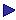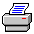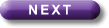Tutorial Contents Tutorial Eight: Relations- Reflexivity - Symmetry -Transitivity - Connectedness - Example of a tableau proof - Eqivalent Relations More Tutorials OneTwoThreeFourFiveSixSymmetry

Rxy is symmetric just if there are no single arrows in its graph; i.e. just if, if the relation holds one way, it also hold the other.

That is:

Rxy is symmetric just if "x"y[Rxy®Ryx]

or, equivalently,

Rxy is symmetric just if ¬\$x\$y[RxyÙ¬Ryx]

Examples: "x is the bother or sister of y"; "x=y".

Rxy is asymmetric just if there are no double arrows in its graph; i.e. just if, if the relation holds one way, it does not hold the other.

That is:

Rxy is asymmetric just if "x"y[Rxy®¬Ryx]

or, equivalently,

Rxy is asymmetric just if ¬\$x\$y[RxyÙRyx]

Examples: "x is the mother of y"; "x>y".

Rxy is non-symmetric just if it is neither symmetric nor asymmetric; i.e. just if there is at least one double arrow and at least one single arrow in its graph.

That is:

Rxy is non-symmetric just if [\$x\$y[RxyÙRyx]Ù\$x\$y[RxyÙ¬Ryx]]

Examples: "x is a brother of y" in suitable domains (remember that John may be Mary's brother without Mary being John's); "x=y2" (in suitable domains).

Again in the empty domain Rxy will be both symmetric and asymmetric, since there will be no arrows at all if there are no dots. But it may be both symmetric and asymmetric in some domains which are not empty, namely if the relation itself is empty: that is, if nothing bears the relation to anything in that domain.

We can express the idea of a relation's being empty like this:

Rxy is empty just if ¬\$x\$yRxy.

Looking at the formal account of symmetry one can see that, if ¬\$x\$yRxy, it follows that ¬\$x\$y[RxyÙ¬Ryx]. Equally, looking at the account of asymmetry one can see that if  ¬\$x\$yRxy, it follows that ¬\$x\$y[RxyÙRyx]. (It does not matter what goes after the "Ù".)

Sometimes we want to prove things about relations by using tableaux. If we are going to do that, we need to express the claim formally. For example, we might want to show that in a non-empty domain, no reflexive relation is asymmetric.

We could express this claim by saying that the following three formulae are inconsistent:

\$xx=x, "xRxx, "x"y[Rxy®¬Ryx].

(The first formula, remember, is a way of saying that there is something: i.e. that the domain is not empty.)

More compactly we could express the claim as a sequent  and it would be natural to use a syntactic sequent if we are going to prove it by a tableau:

\$xx=x, "xRxx, "x"y[Rxy®¬Ryx]|-Print this page